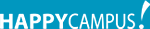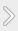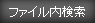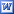#### タグ

レポート理工学力学実験考察ニュートン法則

### 資料紹介

Aim ;
Determine the relationship between mass, force and acceleration.
F = m*a (net force = mass times the acceleration)

2. First, we did the experiment that we change “Force”. We do not change the “Mass”. Only
change the “Force”. We decided to take data of 10N, 30N, 50N, 70N and 90N.
Put right amount of weight on both ends of string and make pulling force to 10N. Hang string with weight on “Atwoods Machine” and hold it.
Next, set “Data Gate” and then make the string let it go. “Atwoods Machine” spins and “Photogate” will automatically send data to “Graphic Calculator”.
And look graph of the data that we took. If the graph look weird, you have to try again.
If graph looks straight, record the points of someplace on the line. (About 4 points)
So you will get the 4 sets of point. (Time and Velocity)

### 資料の原本内容( この資料を購入すると、テキストデータがみえます。 )

--- Newton’s Second Law Lab. -----
Aim ;
Determine the relationship between mass, force and acceleration.
F = m*a (net force = mass times the acceleration)
Materials ;
・Graphic Calculator (Texas Instruments) *With software “Data Gate” installed. ・LAB PRO (Vernier) ・Photogate (Vernier) ・Atwoods Machine (PASCO) ・Stand
・String
・Weight (※We only had “grams” but we use this weight as “kilograms”)
Hypothesis ;
I think if we increase the “Force”, the “Acceleration” also will increase. So I think the g..

## featherの資料(176)

•Relax
•Report image
•Simple and Stylish
•Stylish green
•Vision

### コメント追加

コメントを書込むには会員登録するか、すでに会員の方はログインしてください。